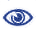# Prague Stringology Conference 2011

A Parameterized Formulation for the Maximum Number of Runs Problem

 Abstract: A parameterized approach to the problem of the maximum number of runs in a string was introduced by Deza and Franek. In the approach referred to as the d-step approach, in addition to the usual parameter the length of the string, the size of the string's alphabet is considered. The behaviour of the function ρd(n), the maximum number of runs over all strings of length n with exactly d distinct symbols, can be handily expressed in the terms of properties of a table referred to as the (d, n - d) table in which ρd(n) is the entry at the dth row and (n - d)th column. The approach leads to a conjectured upper bound ρd(n) ≤ n - d for 2 ≤ d ≤ n. The parameterized formulation shows that the maximum within any column of the (d, n - d) table is achieved on the main diagonal, i.e. for n = 2d, and motivates the investigation of the structural properties of the run-maximal strings of length n bounded by a constant times the size of the alphabet d. We show that ρd(n) = ρn - d(2n - 2d) for 2 ≤ d ≤ n < 2d, ρd(2d) ≤ ρd - 1(2d - 1) + 1 for d > 3, ρd - 1(2d - 1) = ρd - 2(2d - 2) = ρd - 3(2d - 3) for d ≥ 5, and {ρd(n) ≤ n - d for 2 ≤ d ≤ n} ↔ {ρd(9d) ≤ 8d for d ≥ 2}. The results allow for an efficient computational verification of entries in the (d, n - d) table for higher values of n and point to a plausible way of either proving the maximum number of runs conjecture by showing that possible counter-examples on the main diagonal would exhibit an impossible structure, or to discover an unexpected counter-example on the main diagonal of the (d, n - d) table. This approach provides a purely analytical proof of ρd(2d) = d for d ≤ 15 and, using the computational results of ρ2(d + 2) for d = 16, …, 23, a proof of ρd(2d) = d for d ≤ 23.

 Download paper:PostScript PDF BibTeX reference
 Download presentation: